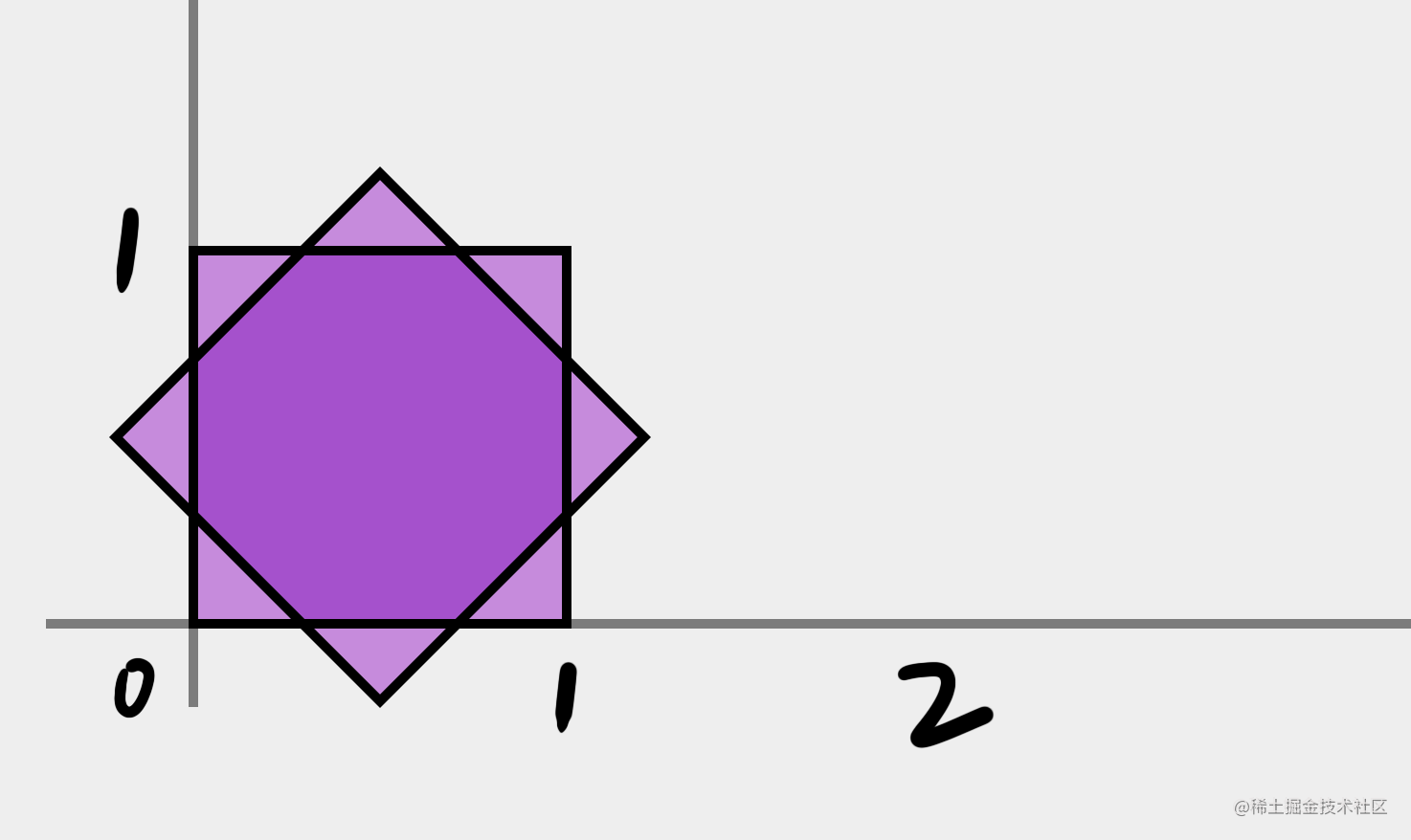# 矩阵乘法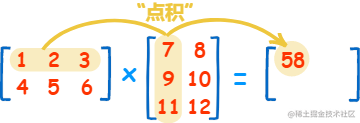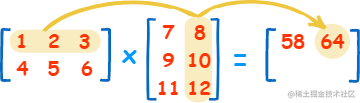1. 当 A 的列数等于 B 的行数时，A 与 B 才可以相乘
2. C 的行数等于 A 的行数，C 的列数等于 B 的列数，即 AC 同高，BC 同宽。
3. C(m,n) = A(m,1) x B(1,n) + A(m,2) x B(2,n) + ...

## 这玩意有什么用？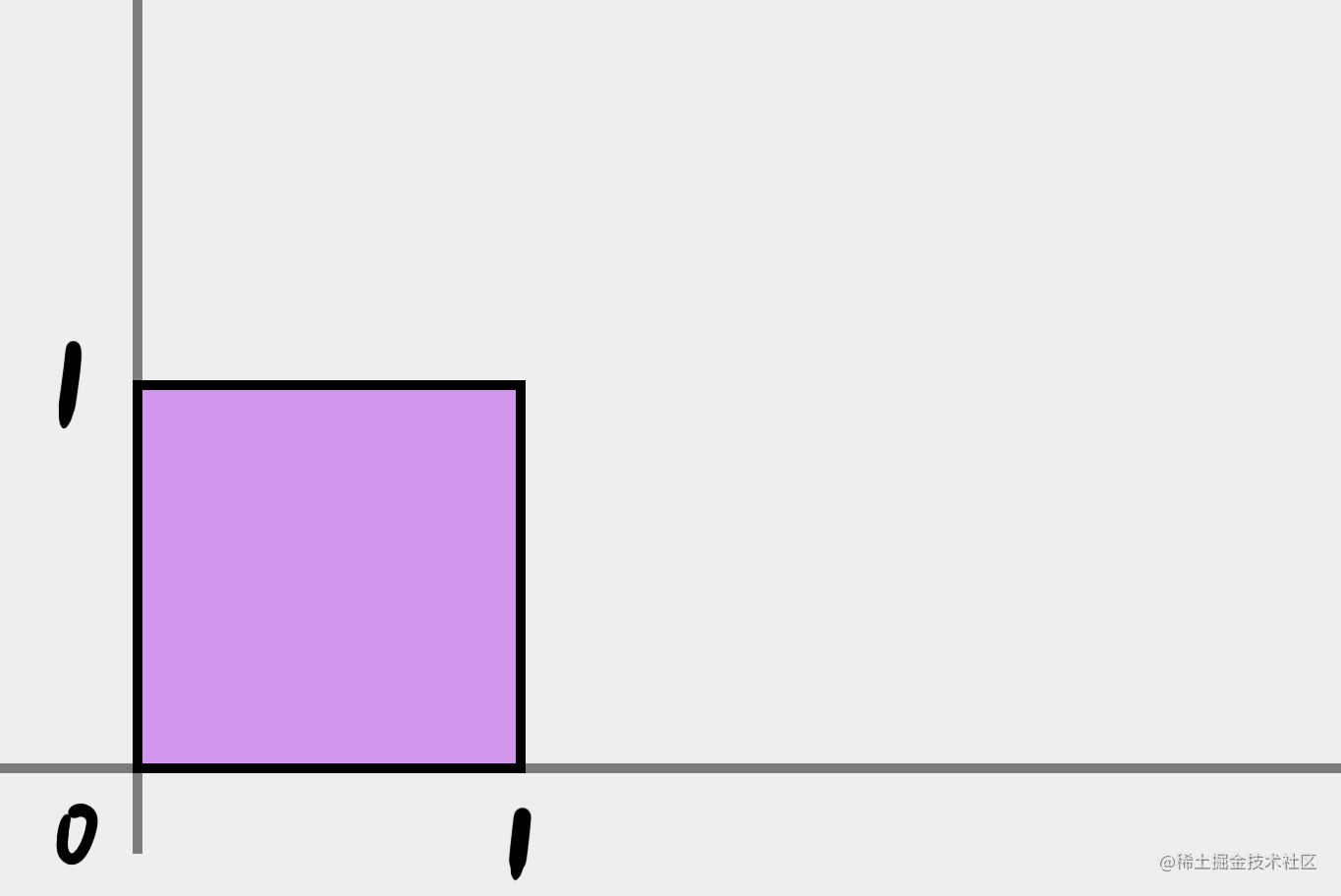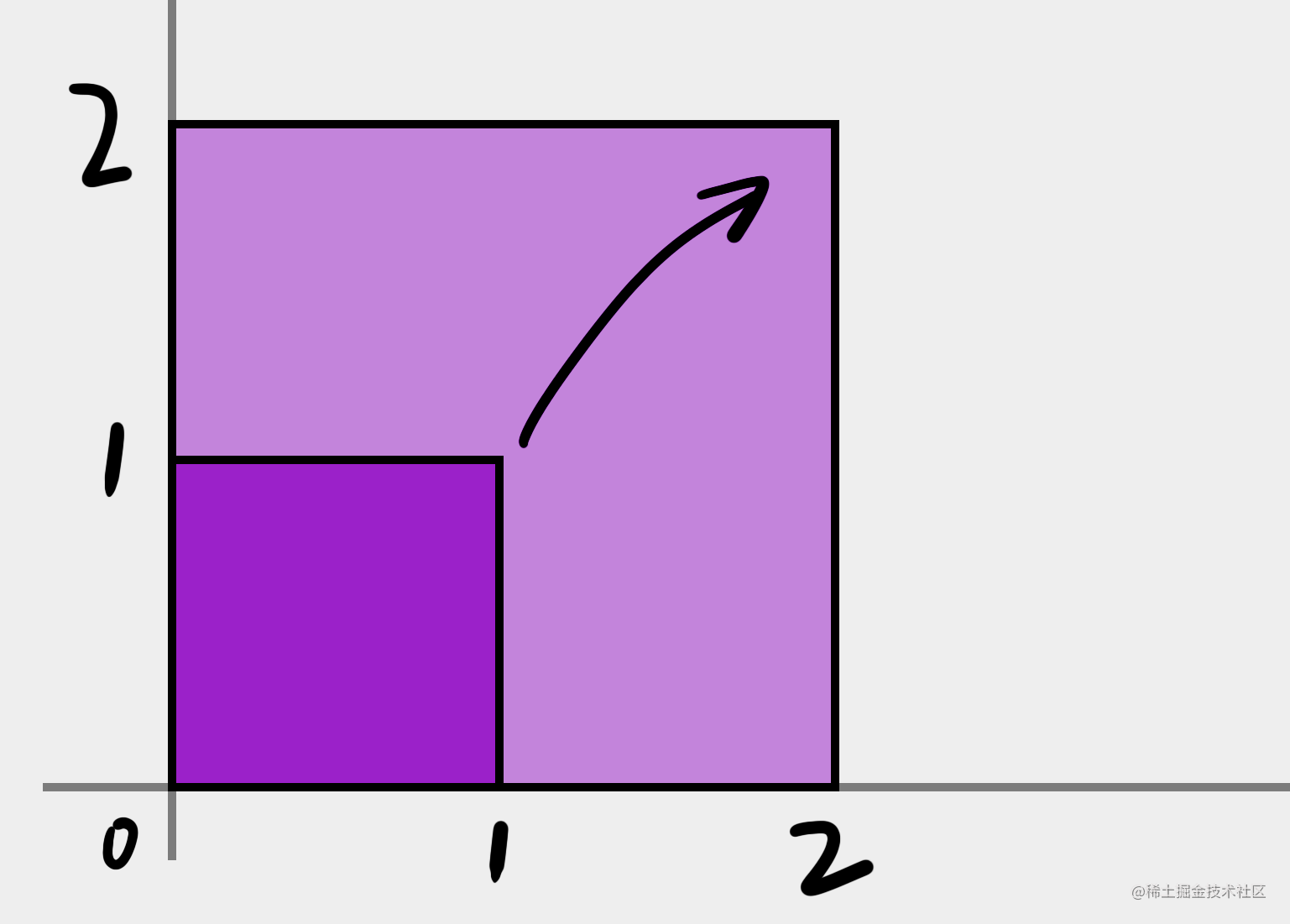$\begin{bmatrix} x & y \end{bmatrix} \begin{bmatrix} 2 & 0\\ 0 & 2 \end{bmatrix} = \begin{bmatrix} 2x+0y & 0x + 2y \end{bmatrix} = \begin{bmatrix} 2x & 2y \end{bmatrix}$

• (1,1) => (2,2)
• (1,0) => (2,0)
• (0,1) => (0,2)
• (0,0) => (0,0)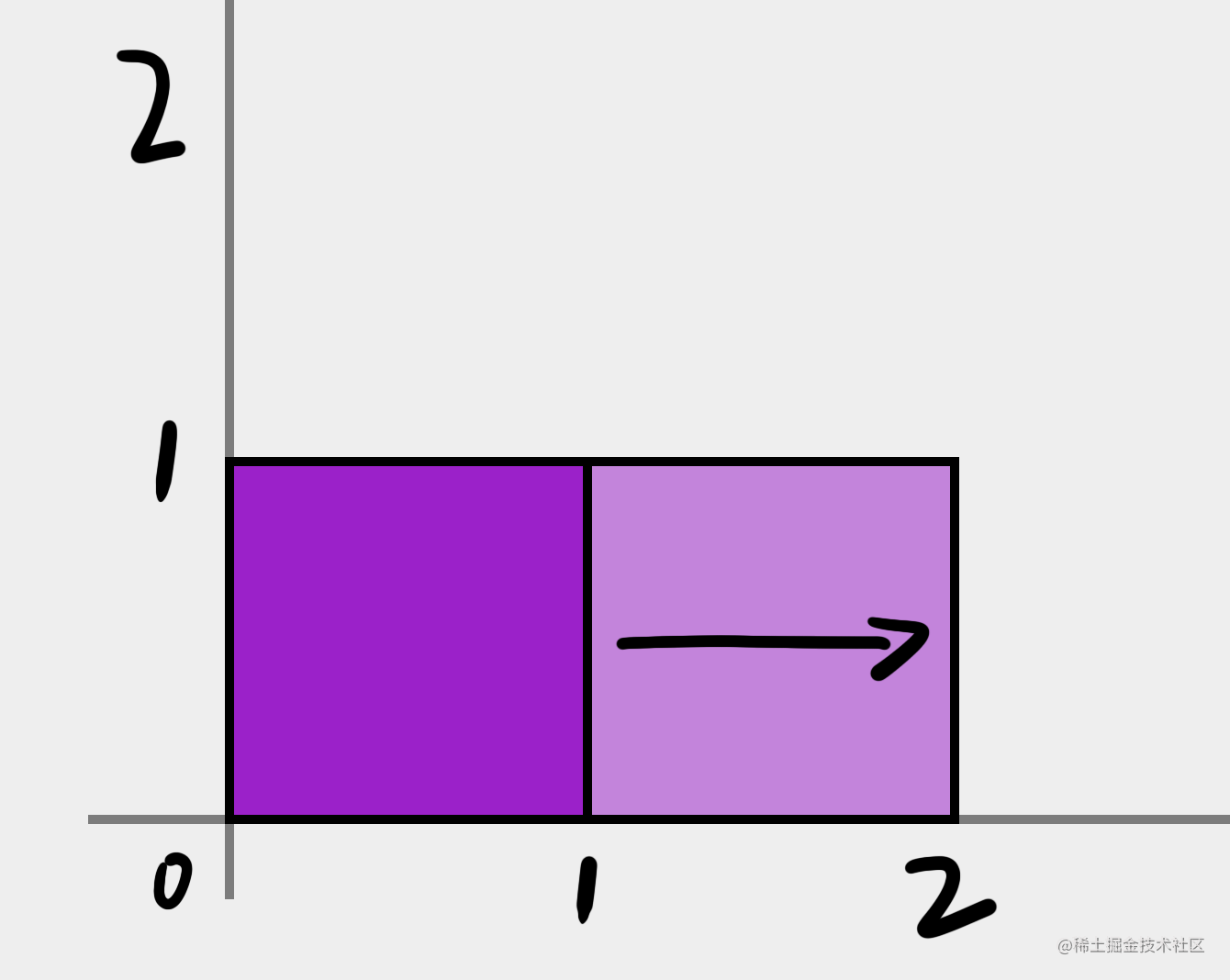$\begin{bmatrix} x & y \end{bmatrix} \begin{bmatrix} 2 & 0\\ 0 & 1 \end{bmatrix} = \begin{bmatrix} 2x & y \end{bmatrix}$

• (1,1) => (2,1)
• (1,0) => (2,0)
• (0,1) => (0,1)
• (0,0) => (0,0)

$\begin{bmatrix} 2表示x的变化 & 0\\ 0 & 1表示y不变 \end{bmatrix}$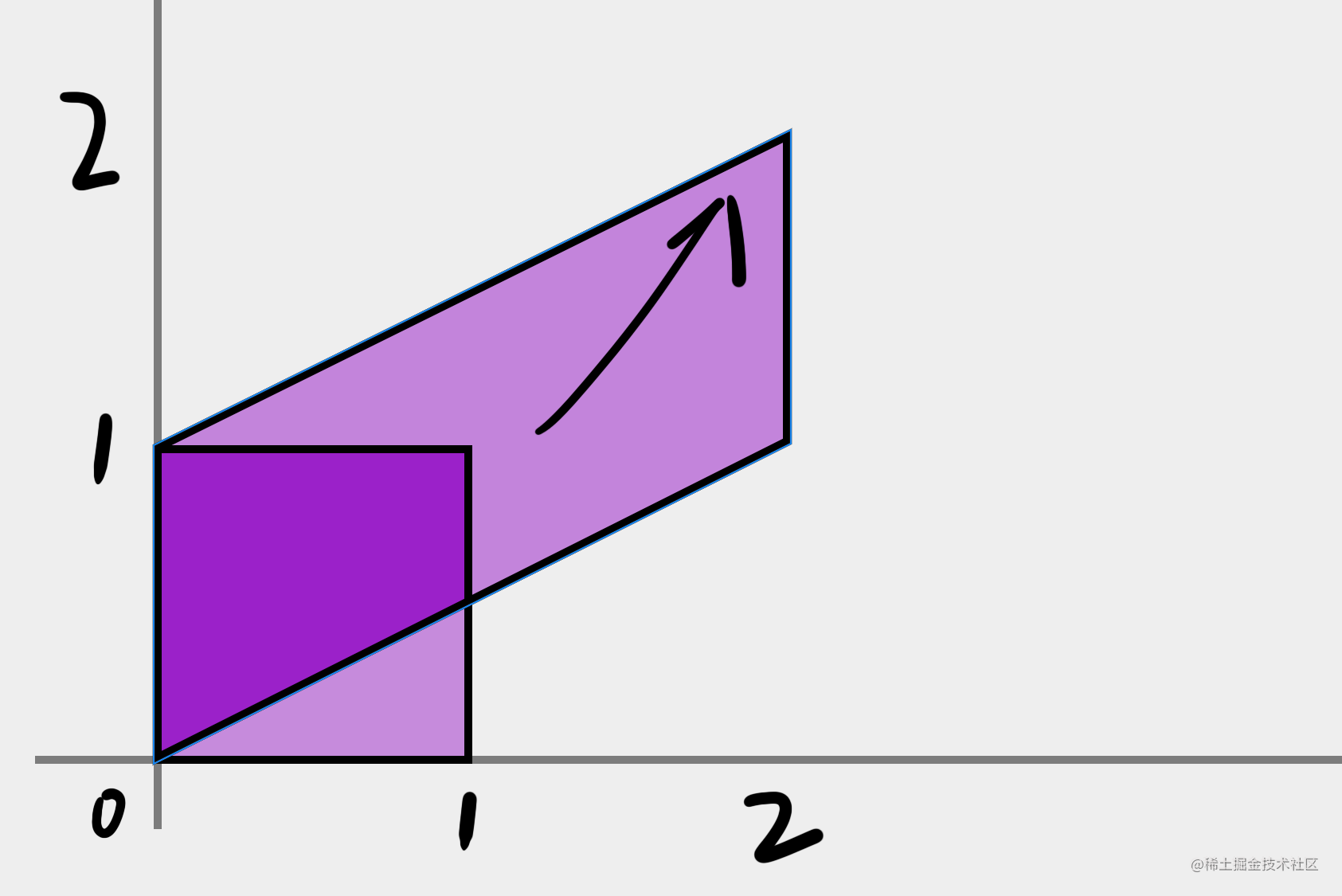$\begin{bmatrix} x & y \end{bmatrix} \begin{bmatrix} 2 & 1\\ 0 & 1 \end{bmatrix} = \begin{bmatrix} 2x & x+y \\ \end{bmatrix}$

• (1,1) => (2,2)
• (1,0) => (2,1)
• (0,1) => (0,1)
• (0,0) => (0,0)

$\begin{bmatrix} x & y \end{bmatrix} \begin{bmatrix} 2 & 0\\ 1 & 1 \end{bmatrix} = \begin{bmatrix} 2x+y & y \\ \end{bmatrix}$

• (1,1) => (3,1)
• (1,0) => (2,0)
• (0,1) => (1,1)
• (0,0) => (0,0)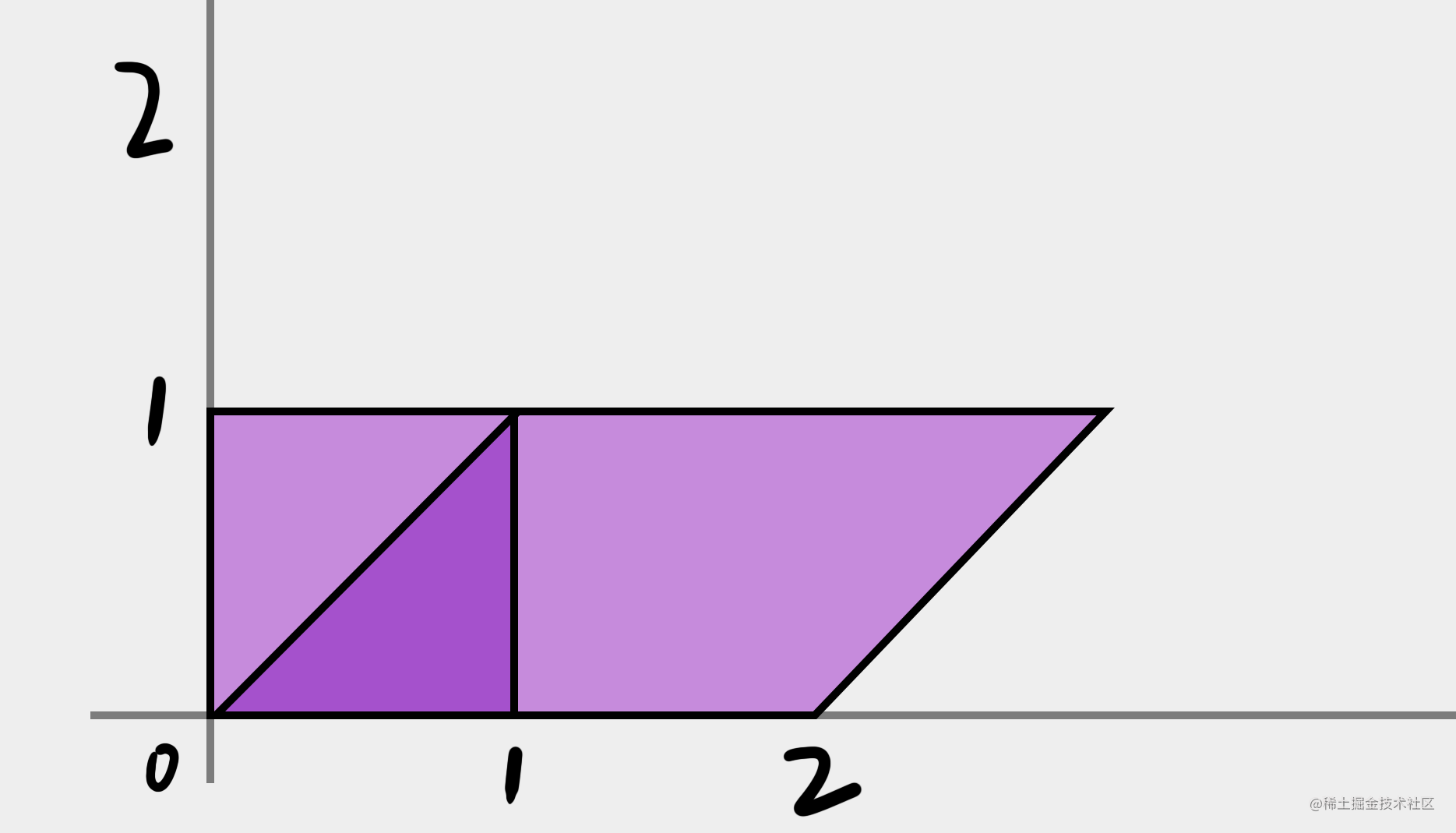$\begin{bmatrix} x在x轴的变化量 & y在x轴的变化量\\ x在y轴的变化量 & y在y轴的变化量 \end{bmatrix}$

$\begin{bmatrix} x=>x轴 & y=>x轴 \\ x=>y轴 & y=>y轴 \end{bmatrix}$

$\begin{bmatrix} x=>x轴 & y=>x轴 \\ x=>y轴 & y=>y轴 \\ x固定增量 & y固定增量 \end{bmatrix}$

$\begin{bmatrix} x & y & 1 \end{bmatrix} \begin{bmatrix} 1 & 0\\ 0 & 1 \\ 10 & 10 \end{bmatrix} = \begin{bmatrix} x+10 & y+10 \\ \end{bmatrix}$

$\begin{bmatrix} x & y & 1 \end{bmatrix} \begin{bmatrix} 1 & 0 & 0\\ 0 & 1 & 0\\ 10 & 10 & 1 \end{bmatrix} = \begin{bmatrix} x+10 & y+10 & 1 \\ \end{bmatrix}$